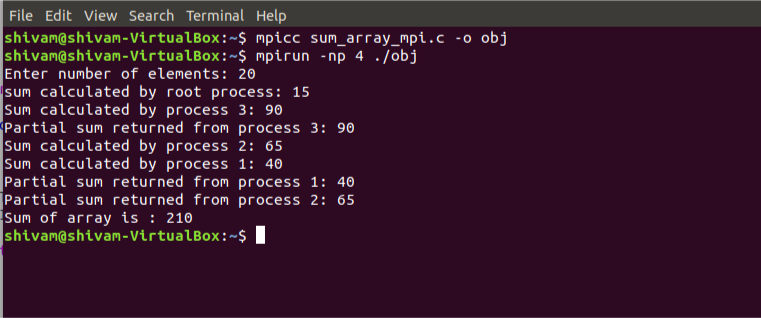# Sum of an array using MPI

• Difficulty Level : Hard
• Last Updated : 28 Jun, 2019

Prerequisite: MPI – Distributed Computing made easy

Message Passing Interface(MPI) is a library of routines that can be used to create parallel programs in C or Fortran77. It allows users to build parallel applications by creating parallel processes and exchange information among these processes.
MPI uses two basic communication routines:

• MPI_Send, to send a message to another process.
• MPI_Recv, to receive a message from another process.

The syntax of MPI_Send and MPI_Recv is:

```int MPI_Send(void *data_to_send,
int send_count,
MPI_Datatype send_type,
int destination_ID,
int tag,
MPI_Comm comm);

int sender_ID,
int tag,
MPI_Comm comm,
MPI_Status *status);
```

To reduce the time complexity of the program, parallel execution of sub-arrays is done by parallel processes running to calculate their partial sums and then finally, the master process(root process) calculates the sum of these partial sums to return the total sum of the array.

Examples:

```Input : {1, 2, 3, 4, 5, 6, 7, 8, 9, 10}
Output : Sum of array is 55

Input : {1, 3, 5, 10, 12, 20, 4, 50, 100, 1000}
Output : Sum of array is 1205
```

Note – You must have MPI installed on your Linux based system for executing the following program. For details to do so, please refer MPI – Distributed Computing made easy

Compile and run the program using following code:

```mpicc program_name.c -o object_file
mpirun -np [number of processes] ./object_file
```

Below is the implementation of the above topic:

 `#include ` `#include ` `#include ` `#include ` ` `  `// size of array ` `#define n 10 ` ` `  `int` `a[] = { 1, 2, 3, 4, 5, 6, 7, 8, 9, 10 }; ` ` `  `// Temporary array for slave process ` `int` `a2; ` ` `  `int` `main(``int` `argc, ``char``* argv[]) ` `{ ` ` `  `    ``int` `pid, np, ` `        ``elements_per_process, ` `        ``n_elements_recieved; ` `    ``// np -> no. of processes ` `    ``// pid -> process id ` ` `  `    ``MPI_Status status; ` ` `  `    ``// Creation of parallel processes ` `    ``MPI_Init(&argc, &argv); ` ` `  `    ``// find out process ID, ` `    ``// and how many processes were started ` `    ``MPI_Comm_rank(MPI_COMM_WORLD, &pid); ` `    ``MPI_Comm_size(MPI_COMM_WORLD, &np); ` ` `  `    ``// master process ` `    ``if` `(pid == 0) { ` `        ``int` `index, i; ` `        ``elements_per_process = n / np; ` ` `  `        ``// check if more than 1 processes are run ` `        ``if` `(np > 1) { ` `            ``// distributes the portion of array ` `            ``// to child processes to calculate ` `            ``// their partial sums ` `            ``for` `(i = 1; i < np - 1; i++) { ` `                ``index = i * elements_per_process; ` ` `  `                ``MPI_Send(&elements_per_process, ` `                         ``1, MPI_INT, i, 0, ` `                         ``MPI_COMM_WORLD); ` `                ``MPI_Send(&a[index], ` `                         ``elements_per_process, ` `                         ``MPI_INT, i, 0, ` `                         ``MPI_COMM_WORLD); ` `            ``} ` ` `  `            ``// last process adds remaining elements ` `            ``index = i * elements_per_process; ` `            ``int` `elements_left = n - index; ` ` `  `            ``MPI_Send(&elements_left, ` `                     ``1, MPI_INT, ` `                     ``i, 0, ` `                     ``MPI_COMM_WORLD); ` `            ``MPI_Send(&a[index], ` `                     ``elements_left, ` `                     ``MPI_INT, i, 0, ` `                     ``MPI_COMM_WORLD); ` `        ``} ` ` `  `        ``// master process add its own sub array ` `        ``int` `sum = 0; ` `        ``for` `(i = 0; i < elements_per_process; i++) ` `            ``sum += a[i]; ` ` `  `        ``// collects partial sums from other processes ` `        ``int` `tmp; ` `        ``for` `(i = 1; i < np; i++) { ` `            ``MPI_Recv(&tmp, 1, MPI_INT, ` `                     ``MPI_ANY_SOURCE, 0, ` `                     ``MPI_COMM_WORLD, ` `                     ``&status); ` `            ``int` `sender = status.MPI_SOURCE; ` ` `  `            ``sum += tmp; ` `        ``} ` ` `  `        ``// prints the final sum of array ` `        ``printf``(``"Sum of array is : %d\n"``, sum); ` `    ``} ` `    ``// slave processes ` `    ``else` `{ ` `        ``MPI_Recv(&n_elements_recieved, ` `                 ``1, MPI_INT, 0, 0, ` `                 ``MPI_COMM_WORLD, ` `                 ``&status); ` ` `  `        ``// stores the received array segment ` `        ``// in local array a2 ` `        ``MPI_Recv(&a2, n_elements_recieved, ` `                 ``MPI_INT, 0, 0, ` `                 ``MPI_COMM_WORLD, ` `                 ``&status); ` ` `  `        ``// calculates its partial sum ` `        ``int` `partial_sum = 0; ` `        ``for` `(``int` `i = 0; i < n_elements_recieved; i++) ` `            ``partial_sum += a2[i]; ` ` `  `        ``// sends the partial sum to the root process ` `        ``MPI_Send(&partial_sum, 1, MPI_INT, ` `                 ``0, 0, MPI_COMM_WORLD); ` `    ``} ` ` `  `    ``// cleans up all MPI state before exit of process ` `    ``MPI_Finalize(); ` ` `  `    ``return` `0; ` `} `

Output:

```Sum of array is 55
```

Below is the snapshot of the processes calculating their partial sums:My Personal Notes arrow_drop_up
Recommended Articles
Page :